## How to limit number of decimal places in formula in Excel?

For example, you sum a range and get a sum value with four decimal places in Excel. You may think of formatting this sum value to one decimal place in the Format Cells dialog box. Actually you can limit the number of decimal places in formula directly. This article is talking about limiting number of decimal places with Format Cells command, and limiting number of decimal places with Round formula in Excel.

#### Limit number of decimal places with Format Cell command in Excel

Normally we can format cells to limit the number of decimal places in Excel easily.

1. Select the cells you want to limit the number of decimal places.

2. Right click the selected cells, and select the Format Cells from the right-clicking menu.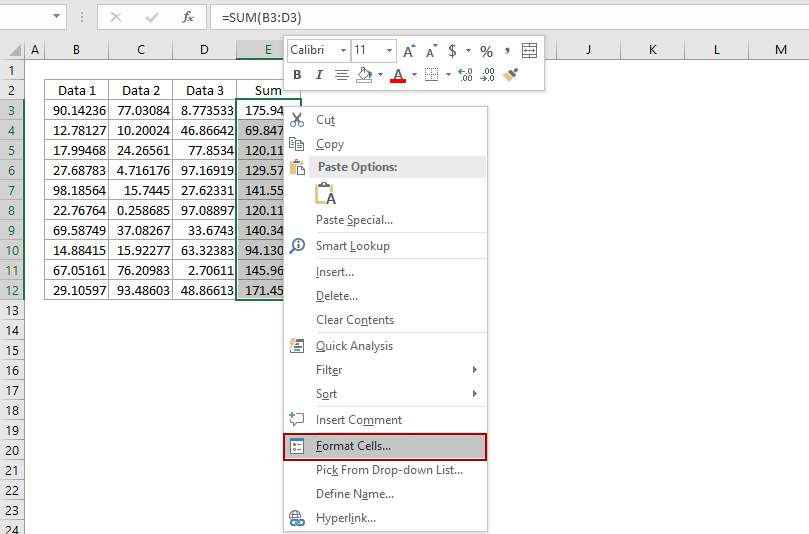3. In the coming Format Cells dialog box, go to the Number tab, click to highlight the Number in the Category box, and then type a number in the Decimal Places box.
For example, if you want to limit only 1 decimal place for selected cells, just type 1 into the Decimal places box. See below screen shot: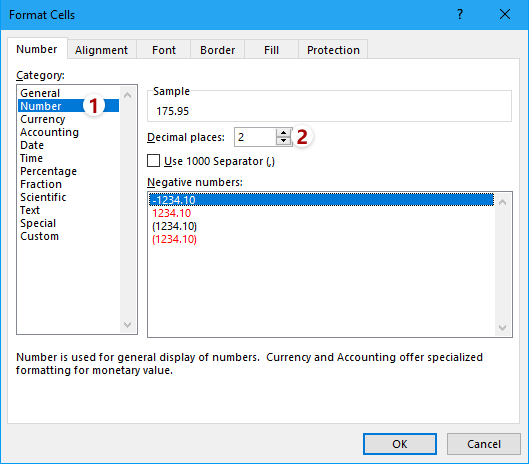4. Click the OK in the Format Cells dialog box. Then you will see all decimals in selected cells are changed to one decimal place.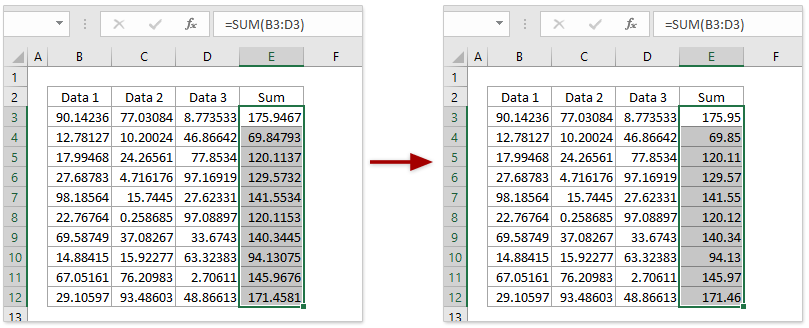Note: After selecting cells, you can click the Increase Decimal button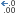or Decrease Decimal button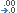directly in the Number group on the Home tab to change the decimal places.

### Easily limit number of decimal places in multiple formulas in Excel

In general, you can use =Round(original_formula, num_digits) to limit the number of decimal places in one formula easily. However, it will be quite tedious and time-consuming to modify multiple formulas one by one manually. Here, use the Operation feature of Kutools for Excel, you can easily limit number of decimal places in multiple formulas easily!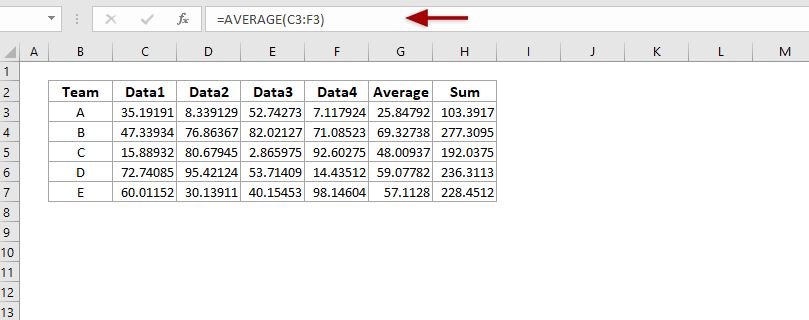Kutools for Excel - Supercharge Excel with over 300 essential tools. Enjoy a full-featured 30-day FREE trial with no credit card required! Get It Now

#### Limit number of decimal places in formulas in Excel

Let's say you calculate the sum of a series of numbers, and you want to limit the number of decimal places for this sum value in the formula, how could you get it done in Excel? You should try the Round function.

The basic Round formula syntax is:

=ROUND(number, num_digits)

And if you want to combine the Round function and other formula, the formula syntax should be changed to

=Round(original_formula, num_digits)

In our case, we want to limit one decimal place for sum value, so we can apply below formula:

=ROUND(SUM(B2:B11),1)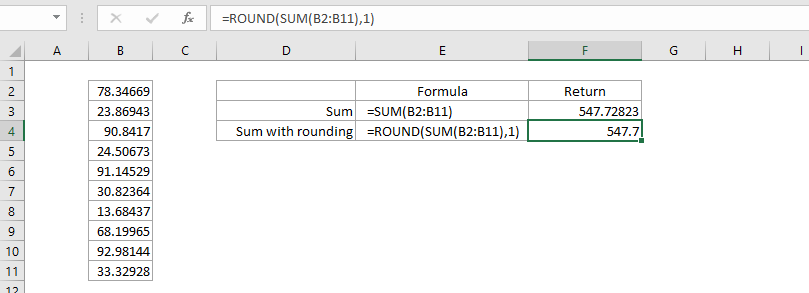#### Limit number of decimal places in multiple formulas in bulk

If you have Kutools for Excel installed, you can apply its Operation feature to modify multiple entered formulas in bulk, such as set rounding in Excel. Please do as follows:

Kutools for Excel- Includes more than 300 handy tools for Excel. Full feature free trial 30-day, no credit card required! Get It Now

1. Select the formula cells whose decimal places you need to limit, and click Kutools > More > Operation.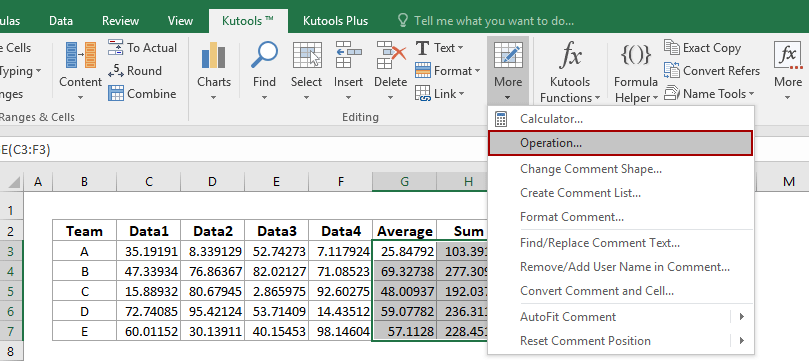2. In the Operation Tools dialog, please click to highlight Rounding in the Operation list box, type the number of decimal places in the Operand section, and check the Create formulas option.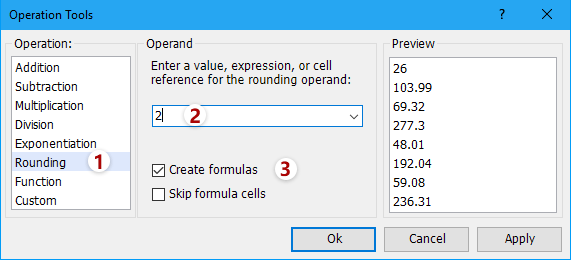3. Click the Ok button.

Now you will see the all formula cells are rounded to the specified decimal places in bulk. See screenshot: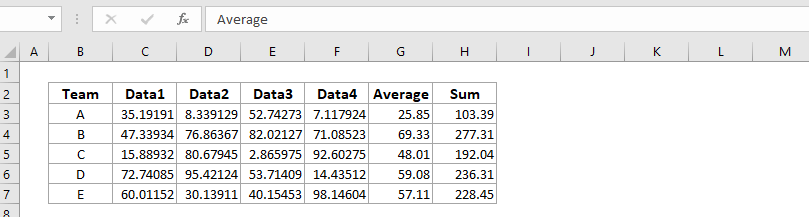Tip: If you need to round down or round up multiple formula cells in bulk, you can set as follows: in the Operation Tools dialog, (1) select Custom in the Operation list box, (2) type =ROUNDUP(?, 2) or =ROUNDDOWN(?, 2) in the Custom section, and (3) check the Create formulas option. See screenshot: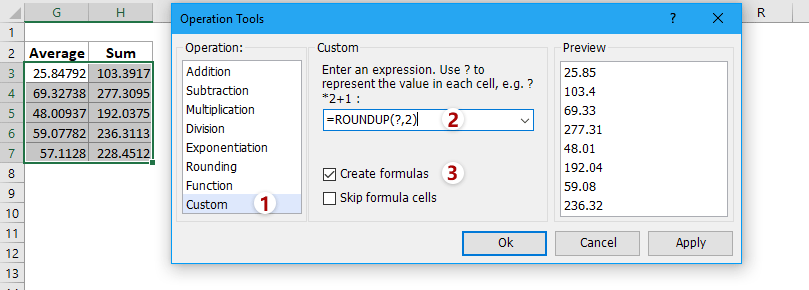#### Easily limit number of decimal places in multiple formulas in Excel

Normally the Decimal feature can decrease the decimal places in cells, but the actual values showing in formula bar don’t change at all. Kutools for Excel’s Round utility can help you round values up/down/to even to specified decimal places easily.

Kutools for Excel- Includes more than 300 handy tools for Excel. Full feature free trial 60-day, no credit card required! Get It Now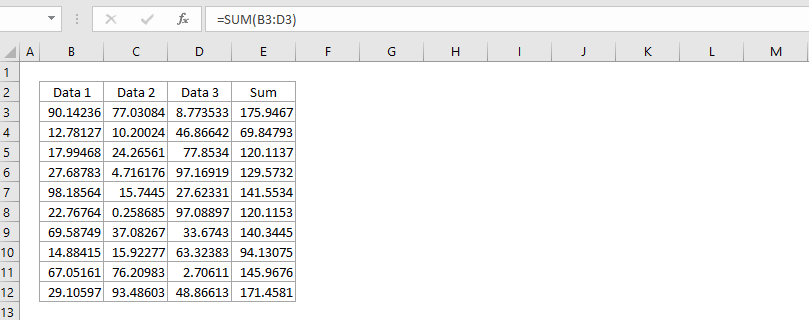This method will round the formula cells to the specified number of decimal places in bulk. However, it will remove formulas from these formula cells and remain the rounding results only.

### Best Office Productivity Tools

 Popular Features: Find, Highlight or Identify Duplicates   |  Delete Blank Rows   |  Combine Columns or Cells without Losing Data   |   Round without Formula ... Super Lookup: Multiple Criteria VLookup  |   Multiple Value VLookup  |   VLookup Across Multiple Sheets   |   Fuzzy Lookup .... Advanced Drop-down List: Quickly Create Drop Down List   |  Dependent Drop Down List   |  Multi-select Drop Down List .... Column Manager: Add a Specific Number of Columns  |  Move Columns  |  Toggle Visibility Status of Hidden Columns  |  Compare Ranges & Columns ... Featured Features: Grid Focus   |  Design View   |   Big Formula Bar   |  Workbook & Sheet Manager   |  Resource Library (Auto Text)   |  Date Picker   |  Combine Worksheets   |  Encrypt/Decrypt Cells   |  Send Emails by List   |  Super Filter   |   Special Filter (filter bold/italic/strikethrough...) ... Top 15 Toolsets:  12 Text Tools (Add Text, Remove Characters, ...)   |   50+ Chart Types (Gantt Chart, ...)   |   40+ Practical Formulas (Calculate age based on birthday, ...)   |   19 Insertion Tools (Insert QR Code, Insert Picture from Path, ...)   |   12 Conversion Tools (Numbers to Words, Currency Conversion, ...)   |   7 Merge & Split Tools (Advanced Combine Rows, Split Cells, ...)   |   ... and more

Supercharge Your Excel Skills with Kutools for Excel, and Experience Efficiency Like Never Before. Kutools for Excel Offers Over 300 Advanced Features to Boost Productivity and Save Time.  Click Here to Get The Feature You Need The Most...#### Office Tab Brings Tabbed interface to Office, and Make Your Work Much Easier

• Enable tabbed editing and reading in Word, Excel, PowerPoint, Publisher, Access, Visio and Project.
• Open and create multiple documents in new tabs of the same window, rather than in new windows.
• Increases your productivity by 50%, and reduces hundreds of mouse clicks for you every day!No ratings yet. Be the first to rate!
This comment was minimized by the moderator on the site
this cnu
am using excel fo milk calculations

in 1 crate having 12/lit of milk.
if customar take 12/lit in total it shows 1 using,(=number1/number2)
if customar take 24/ in total it shows 2 using,(=number1/number2)
if customar take 36/ total it shows 3using,(=number1/number2)
but some customar take 15 or 22 or 35

if customar take 15 in total it shows 1.3
if customar take 22 in total it shows 1.10
if customar take35 in total it shows 2.11

i need formula for above calculation
This comment was minimized by the moderator on the site
try this:
=roundup(number1/number2,0)
This comment was minimized by the moderator on the site
'95,954,691.3389700060
When a put this data on excel, and quit the ' to turn it a number, excel, trucante the number after 5 decimal. The new number is 95,954,691.33897, but I need 10 decimals. How to resolve this?
This comment was minimized by the moderator on the site
Try this:

=TEXT(95954691.3389100060,"00,000,000.0000000000")
This comment was minimized by the moderator on the site
Ok, here is one I really need help with since it would ease my job a lot. I have a table where i need most of my cells to have 2 decimals. One of the columns (lets say C) has to be A multiplied by B, but with a little text following the result of the multiplication, hence i gave it a:
A*B & "text" formula
My problem is that if result of A*B is 3 or 3.1 it will appear just like that: "3 text" or "3.1 text", but i really need it as "3.00 text"/ "3.10text".
Any tips?
This comment was minimized by the moderator on the site
Try this:

=TEXT(A*B,"0.00")&" text"
This comment was minimized by the moderator on the site
I want to set a limit whereas 0.60 is the threshold if the decimal reaches 0.60 it will automatically count as 1 and reset into decimal. The decimal represent the minutes and the whole number represent the hours. I'm presenting my data as numbers instead of timevalue. Hope anyone can help
This comment was minimized by the moderator on the site
Unfortunately it is not working if you concatenate:

I want to combine two cells A and B with many digits to A (B) with onlytwo digits.

I tried like that:
=CONCATENATE((ROUND(C4,1))," (",(Round(D4,1)),")")

It doesn't work and shows only the formula.
Can anyone help?
This comment was minimized by the moderator on the site
Hi yvonne Fabian,
Your formula works well in my Excel 2016.
This comment was minimized by the moderator on the site
how to deal with two decimal points e.g. 138.4622.472, 137.1416.113 etc. any way to get rid of .472 and .113 and so on
This comment was minimized by the moderator on the site
John, to round to 0 decimals, try use ROUND(CELL,0) and to round DOWN to the number, use INT (integer).
ROUND(12.5,0) = 13 ; INT(12.5) = 12
for the same but not to whole number but e.g. tenths, first multiply the baste number by 10, then ROUND/INT and then devide back by ten.
This comment was minimized by the moderator on the site
Here we are inserting the values in one cell(A1) and applying the formula in another cell(D1) (for example) and in that cell(D1) only we are getting the output , but I want to apply the formula in cell(A1) only so I can get the output in that cell (A1).
If I am doing this then I am not getting any output in the cell (A1).
This comment was minimized by the moderator on the site
Hi Narsingh,
Kutools for Excel provides an Operation tools which can custom formula to selected cells. This feature may solve your problem.
This comment was minimized by the moderator on the site
Here we are inserting the values in one cell(A1) and applying the formula in another cell(D1) (for example) and in that cell(D1) only we are getting the output , but I want to apply the formula in cell(A1) only so I can get the output in that cell (A1).
If I am doing this then I am not getting any output in the cell (A1).